# Basic Probability¶

• A number between $0$ and $1$.
• A statement about certainty / uncertainty.
• $1$ is complete certainty something is the case.
• $0$ is complete certainty something is not the case.

## Conditional Probability¶

Conditional probability is a measure of the probability of an event occurring given that another event has (by assumption, presumption, assertion or evidence) occurred.

Given two events $A$ and $B$, with the unconditional probability of $B$ (that is, of the event $B$ occurring) being greater than zero, $P(B) > 0$, the conditional probability of $A$ given $B$ is defined as the quotient of the probability of the joint of events $A$ and $B$, and the probability of $B$:

$$P(A \mid B) = \frac{P(A \cap B)}{P(B)}$$

Where $P(A \cap B)$ is the probability that both events $A$ and $B$ occur.

Mathematical notation:

• $\mid$ = given
• $P$( n_visitors = 13) is a probability
• $P$( n_visitors ) is a probability distribution
• $P$( n_visitors = 13 | prop_clicks = 10%) is a conditional probability
• $P$( n_visitors | prop_clicks = 10%) is a conditional probability distribution

## Union and Intersection¶

Union: The union of $2$ sets $A$ and $B$ is denoted by $A \cup B$. This is the set of all distinct elements that are in $A$ or $B$. A useful way to remember the symbol is $\cup$. We can define the union of a collection of sets, as the set of all distinct elements that are in any of these sets.

Intersection: The intersection of $2$ sets $A$ and $B$ is denoted by $A \cap B$. This is the set of all distinct elements that are in both $A$ and $B$. A useful way to remember the symbol is $\cap$. We define the intersection of a collection of sets, as the set of all distinct elements that are in all of these sets.

Example: If $A = \{ 1, 3, 5, 7, 9 \}$ and $B = \{ 2, 3, 5, 7 \}$ what are $A \cup B$ and $A \cap B$ ?

We have:

$$A \cup B = \{ 1, 2, 3, 5, 7, 9 \}$$$$A \cap B = \{ 3, 5, 7 \}$$

## Rule of Sum¶

The probability rule of sum gives the situations in which the probability of a union of events can be calculated by summing probabilities together. It is often used on mutually exclusive events, meaning events that cannot both happen at the same time.

Let $A$ and $B$ be mutually exclusive events. Then, the probability of the union of those events is:

$$P(A \cup B) = P(A) + P(B)$$

## Rule of Product¶

The rule of product is a guideline as to when probabilities can be multiplied to produce another meaningful probability. Specifically, the rule of product is used to find the probability of an intersection of events:

Let $A$ and $B$ be independent events. Then,

$$P(A \cap B) = P(A) \times P(B)$$

## Bayesian inference in a nutshell¶

Bayesian inference is a method for figuring out unobservable quantities given known facts that uses probability to describe the uncertainty over what the values of the unknown quantities could be. The role of probability distributions in Bayesian data analysis is to represent uncertainty, and the role of Bayesian inference is to update probability distributions to reflect what has been learned from data.

### Bayes' theorem formula:¶

$$P(A \mid B) = \frac{P(B \mid A) \times P(A)}{P(B)}$$

where $A$ and $B$ are events and $P(B) \neq 0$.

• $P(A\mid B)$ is a conditional probability: the likelihood of event $A$ occurring given that $B$ is true.

• $P(B\mid A)$ is also a conditional probability: the likelihood of event $B$ occurring given that $A$ is true.

• $P(A)$ and $P(B)$ are the probabilities of observing $A$ and $B$ respectively; they are known as the marginal probability.

#### Bayes' theorem given parameters and data¶

$$P( \theta \mid D) = \frac{P(D \mid \theta) \times P(\theta)}{\sum P(D \mid \theta) \times P(\theta)}$$

Where,

• $\theta$ is a parameter combination,
• $D$ is the data,
• $P(D \mid \theta)$ is the likelihood,
• $P(\theta)$ is the prior,
• $P( \theta \mid D)$ is the probability of different parameter values given the data.

## Imports & Libraries¶

In :
options(warn = -1)
library(ggplot2)
library(ggjoy)
library(ggExtra)
library(devtools)
library(magrittr)
library(lattice)
library(multipanelfigure)
library(purrr)
library(dplyr)
library(forcats)
library(BEST)


# Coin flips with prop_model¶

The function prop_model implements a Bayesian model that assumes that:

• The data is a vector of successes and failures represented by 1s and 0s.
• There is an unknown underlying proportion of success.
• Prior to being updated with data any underlying proportion of success is equally likely.
In :
prop_model <- function(data = c(), prior_prop = c(1, 1), n_draws = 10000,
show_plot = TRUE)
{
data <- as.logical(data)
proportion_success <- c(0, seq(0, 1, length.out = 100), 1)
data_indices <- round(seq(0, length(data), length.out = min(length(data) +
1, 20)))
post_curves <- map_dfr(data_indices, function(i) {
value <- ifelse(i == 0, "Prior", ifelse(data[i], "Success",
"Failure"))
label <- paste0("n=", i)
probability <- dbeta(proportion_success, prior_prop +
sum(data[seq_len(i)]), prior_prop + sum(!data[seq_len(i)]))
probability <- probability/max(probability)
data_frame(value, label, proportion_success, probability)
})
post_curves$label <- fct_rev(factor(post_curves$label, levels = paste0("n=",
data_indices)))
post_curves$value <- factor(post_curves$value, levels = c("Prior",
"Success", "Failure"))
p <- ggplot(post_curves, aes(x = proportion_success, y = label,
height = probability, fill = value)) + geom_joy(stat = "identity",
color = "white", alpha = 0.8, panel_scaling = TRUE, size = 1) +
scale_y_discrete("", expand = c(0.01, 0)) + scale_x_continuous("Underlying proportion of success") +
scale_fill_manual(values = hcl(120 * 2:0 + 15, 100, 65),
name = "", drop = FALSE, labels = c("Prior   ", "Success   ",
"Failure   ")) + theme_light(base_size = 18) +
theme(legend.position = "top")
if (show_plot) {
print(p)
}
invisible(rbeta(n_draws, prior_prop + sum(data), prior_prop +
sum(!data)))
}


Assume you just flipped a coin four times and the result was heads, tails, tails, heads. If you code heads as a success and tails as a failure then the following Rcodes runs prop_model with this data:

In :
data <- c(1, 0, 0, 1)


The output of prop_model is a plot showing what the model learns about the underlying proportion of success from each data point in the order you entered them. At n=0 there is no data, and all the model knows is that it's equally probable that the proportion of success is anything from 0% to 100%. At n=4 all data has been added, and the model knows a little bit more.

In :
prop_model(data)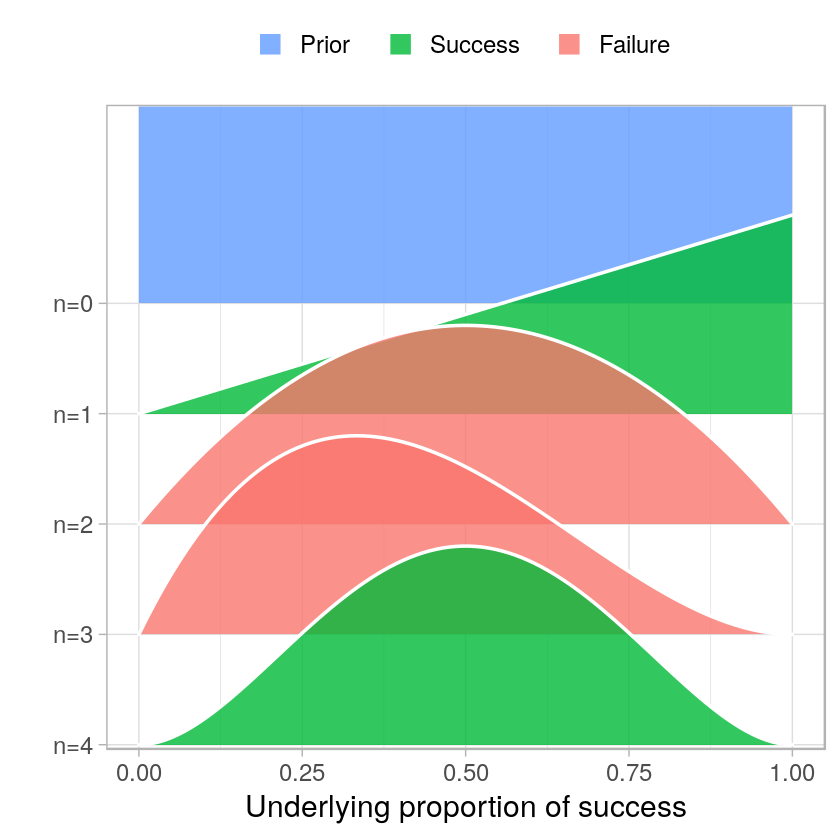If we really were interested in the underlying proportion of heads of this coin then prop_model isn't particularly useful. Since it assumes that any underlying proportion of success is equally likely prior to seeing any data it will take a lot of coin flipping to convince prop_model that the coin is fair. This model is more appropriate in a situation where we have little background knowledge about the underlying proportion of success.

Let's say the zombie apocalypse is upon us and we have come up with a new experimental drug to cure zombieism. We have no clue how effective it's going to be, but when we gave it to 13 zombies two of them turned human again.

In :
# Update the data and rerun prop_model
data = c(0, 0, 0, 0, 0, 0, 0, 0, 0, 0, 0, 1, 1)
prop_model(data)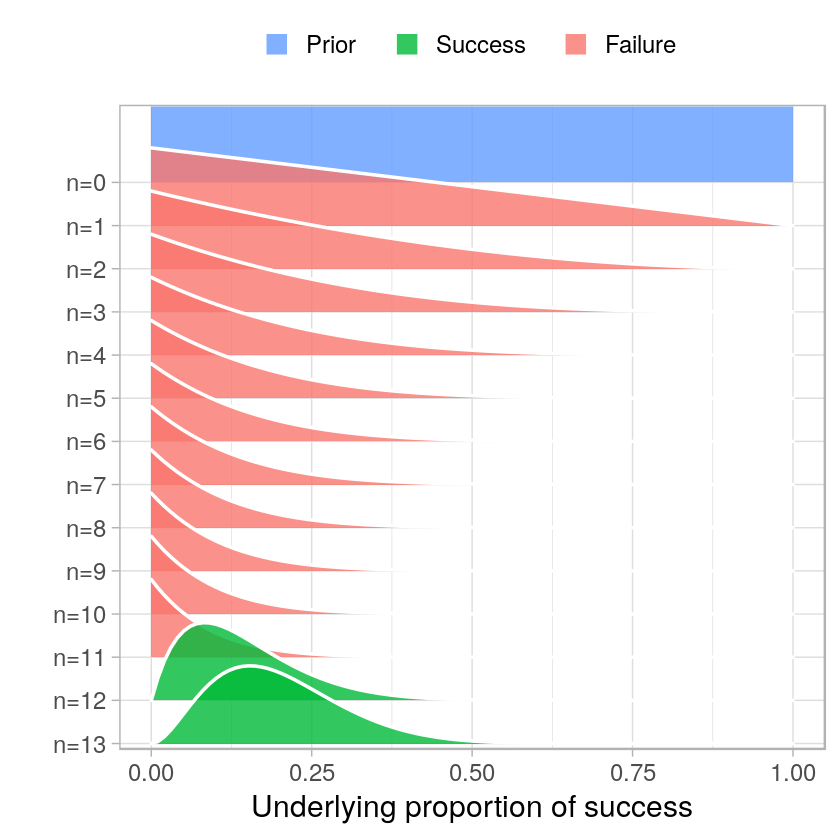## Priors & Posteriors¶

• A prior is a probability distribution that represents what the model knows before seeing the data.
• A posterior is a probability distribution that represents what the model knows after having seen the data.

## Looking at samples from prop_model¶

Here again is the prop_model function which has been given the data from our zombie experiment where two out of 13 zombies got cured. In addition to producing a plot, prop_model also returns a large random sample from the posterior over the underlying proportion of success.

In :
data = c(1, 0, 0, 1, 0, 0, 0, 0, 0, 0, 0, 0, 0)

# Extract and explore the posterior
posterior <- prop_model(data)

1. 0.245610927989934
2. 0.327120493172801
3. 0.258294850397774
4. 0.231941105366075
5. 0.138591625358127
6. 0.128583997285671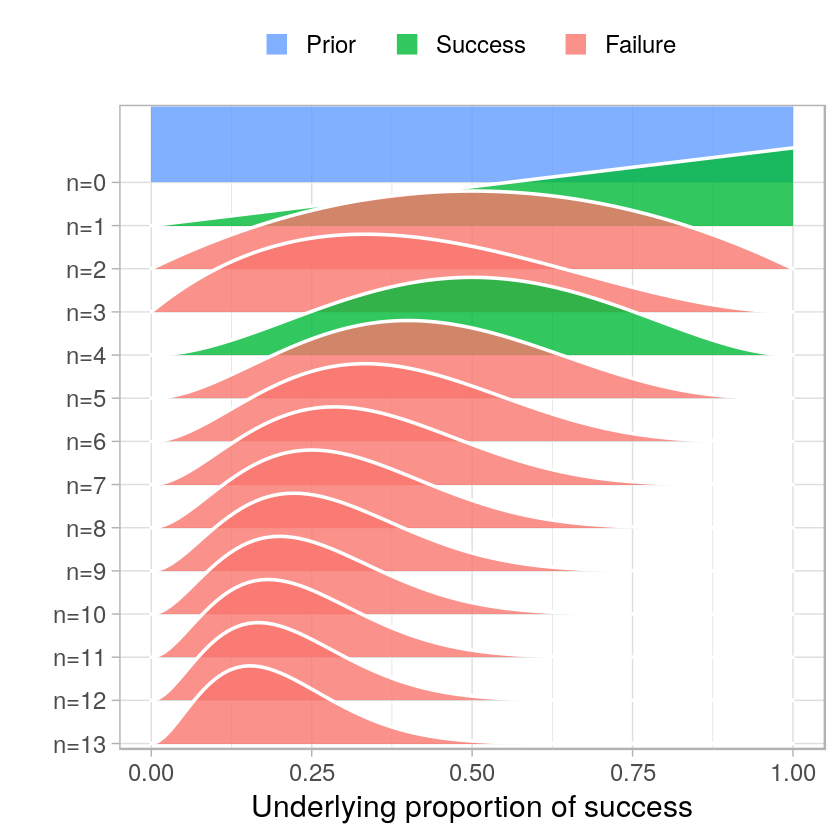Looking at these first few samples confirms what is already shown in the plot: That the underlying proportion of cured zombies is likely somewhere between 5% and 50%. But these were just the first six samples in posterior which currently contain 10,000 samples (the default of prop_model).

In :
hist(posterior, breaks = 30, xlim = c(0, 1), col = "lightgreen")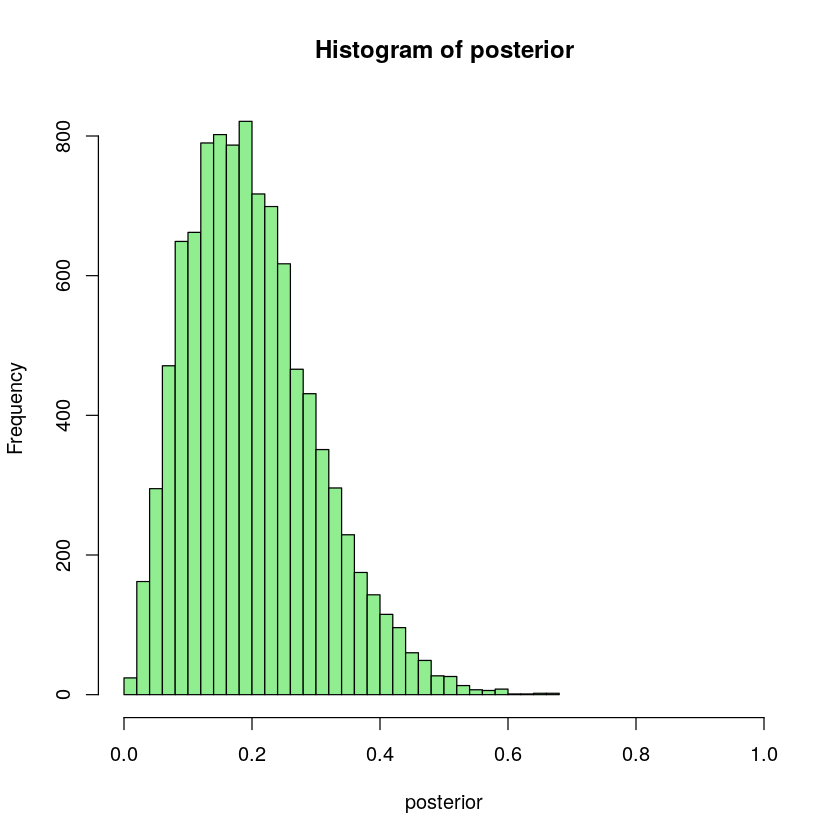## Summarizing the zombie drug experiment¶

The point of working with samples from a probability distribution is that it makes it easy to calculate new measures of interest.

A point estimate is a single number used to summarize what's known about a parameter of interest. It can be seen as a "best guess" of the value of the parameter. A commonly used point estimate is the median of the posterior. It's the midpoint of the distribution, and it's equally probable for the parameter value to be larger than the median as it is to be smaller than it.

In :
# Calculate some measures of interest using the posterior
median(posterior)

0.188772165723979

So, a best guess is that the drug would cure around 19% of all zombies. Another common summary is to report an interval that includes the parameter of interest with a certain probability. This is called a credible interval (CI). With a posterior represented as a vector of samples you can calculate a CI using the quantile() function.

In :
# Calculate a 90% credible interval
quantile(posterior, c(0.05, 0.95))

5%
0.0608266154923048
95%
0.386458729093519

According to the credible interval, there is a 90% probability that the proportion of zombies the drug would cure is between 6% and 39%. (Here we have to be careful to remember that the percentage of cured zombies and the percentage of probability are two different things.)

Now, there is a rival zombie laboratory that is also working on a drug. They claim that they are certain that their drug cures 7% of the zombies it's administered to. Can we calculate how probable it is that our drug is better?

In :
# use sum to count how many samples in posterior that are larger than 7%.
sum(posterior > 0.07)

9310

To turn this count into a probability we now need to normalize it, that is, divide it by the total number of samples in posterior

In :
# Calculate the probability
sum(posterior > 0.07) / length(posterior)

0.931

It seems there is a large probability (93%) that our zombie drug is better!

#### The result in a journal¶

Given the data of 2 cured and 11 relapsed zombies, and using the Baysian model describes before, there is a 90% probability that our drug cures between 6% and 39% of treated zombies. Further, there is a 93% probability that our drug cures zombies at a higher rate than the current state of the art drug.

# generative model:¶

Any type of computer program, mathematical expression, or a set of rules that you can feed fixed parameter values, and that you can use to generate simulated data.

## Take a generative model for a spin¶

Assume the underlying proportion of success of curing a zombie is 42% and that you administer the drug to 100 zombies.

In :
# The generative zombie drug model

# Set parameters
prop_success <- 0.42
n_zombies <- 100

# Simulating data
data <- c()
for(zombie in 1:n_zombies) {
data[zombie] <- runif(1, min = 0, max = 1) < prop_success
}
data <- as.numeric(data)
data

1. 1
2. 1
3. 0
4. 0
5. 0
6. 0
7. 0
8. 0
9. 0
10. 0
11. 0
12. 0
13. 1
14. 0
15. 1
16. 0
17. 1
18. 1
19. 0
20. 0
21. 1
22. 1
23. 1
24. 1
25. 1
26. 1
27. 1
28. 0
29. 0
30. 1
31. 1
32. 0
33. 1
34. 0
35. 1
36. 0
37. 0
38. 0
39. 1
40. 1
41. 1
42. 1
43. 0
44. 0
45. 1
46. 0
47. 0
48. 0
49. 1
50. 1
51. 0
52. 1
53. 0
54. 0
55. 1
56. 1
57. 1
58. 1
59. 0
60. 0
61. 1
62. 0
63. 0
64. 0
65. 1
66. 0
67. 0
68. 0
69. 1
70. 1
71. 0
72. 0
73. 0
74. 0
75. 1
76. 1
77. 0
78. 0
79. 0
80. 0
81. 1
82. 0
83. 0
84. 1
85. 0
86. 1
87. 0
88. 0
89. 0
90. 0
91. 1
92. 0
93. 0
94. 0
95. 1
96. 1
97. 1
98. 0
99. 0
100. 1
In :
# Count cured zombies
data <- sum(data == 1)
data

43

# Take the binomial distribution for a spin¶

It turns out that the generative model we ran last has a name. It's called the binomial process or the binomial distribution. In R you can use the rbinom function to simulate data from a binomial distribution. The rbinom function takes three arguments:

• n The number of times you want to run the generative model
• size The number of trials. (For example, the number of zombies you're giving the drug.)
• prob The underlying proportion of success as a number between 0.0 and 1.0.
In :
# Try out rbinom
rbinom(n = 1, size = 100, prob = 0.42)

45
In :
# Change the parameters to run the simulation 200 times
rbinom(n = 200, size = 100, prob = 0.42)

1. 41
2. 48
3. 33
4. 42
5. 38
6. 40
7. 42
8. 40
9. 50
10. 41
11. 40
12. 42
13. 46
14. 36
15. 53
16. 37
17. 38
18. 38
19. 42
20. 36
21. 39
22. 36
23. 38
24. 41
25. 33
26. 44
27. 43
28. 41
29. 36
30. 44
31. 49
32. 38
33. 44
34. 40
35. 34
36. 39
37. 37
38. 35
39. 37
40. 47
41. 45
42. 42
43. 37
44. 39
45. 43
46. 43
47. 37
48. 42
49. 47
50. 38
51. 36
52. 41
53. 45
54. 37
55. 34
56. 44
57. 45
58. 47
59. 41
60. 36
61. 45
62. 35
63. 43
64. 34
65. 40
66. 40
67. 40
68. 43
69. 44
70. 50
71. 41
72. 35
73. 49
74. 35
75. 44
76. 42
77. 42
78. 43
79. 44
80. 43
81. 46
82. 42
83. 40
84. 40
85. 42
86. 46
87. 44
88. 46
89. 38
90. 40
91. 48
92. 41
93. 46
94. 43
95. 41
96. 43
97. 52
98. 33
99. 47
100. 35
101. 43
102. 47
103. 40
104. 35
105. 40
106. 44
107. 44
108. 37
109. 35
110. 49
111. 33
112. 41
113. 45
114. 49
115. 41
116. 49
117. 52
118. 44
119. 43
120. 45
121. 36
122. 38
123. 46
124. 42
125. 43
126. 42
127. 43
128. 35
129. 45
130. 41
131. 40
132. 42
133. 45
134. 46
135. 40
136. 49
137. 45
138. 37
139. 39
140. 37
141. 36
142. 40
143. 41
144. 52
145. 44
146. 35
147. 48
148. 38
149. 40
150. 38
151. 45
152. 44
153. 40
154. 33
155. 44
156. 39
157. 38
158. 34
159. 39
160. 35
161. 42
162. 37
163. 44
164. 47
165. 30
166. 37
167. 45
168. 40
169. 39
170. 46
171. 45
172. 43
173. 42
174. 37
175. 44
176. 38
177. 47
178. 46
179. 41
180. 47
181. 48
182. 37
183. 48
184. 47
185. 45
186. 37
187. 40
188. 47
189. 51
190. 42
191. 39
192. 52
193. 50
194. 40
195. 39
196. 47
197. 47
198. 48
199. 50
200. 35

# How many visitors could your site get?¶

To get more visitors to your website you are considering paying for an ad to be shown 100 times on a popular social media site. According to the social media site, their ads get clicked on 10% of the time.

Assume that 10% is a reasonable number, and assume that the binomial distribution is a reasonable generative model for how people click on ads since it is a binary decision.

In :
# Fill in the parameters
n_samples <- 100000
proportion_clicks <- 0.10

n_visitors <- rbinom(n_samples, size = n_ads_shown, prob = proportion_clicks)

# Visualize n_visitors
hist(n_visitors)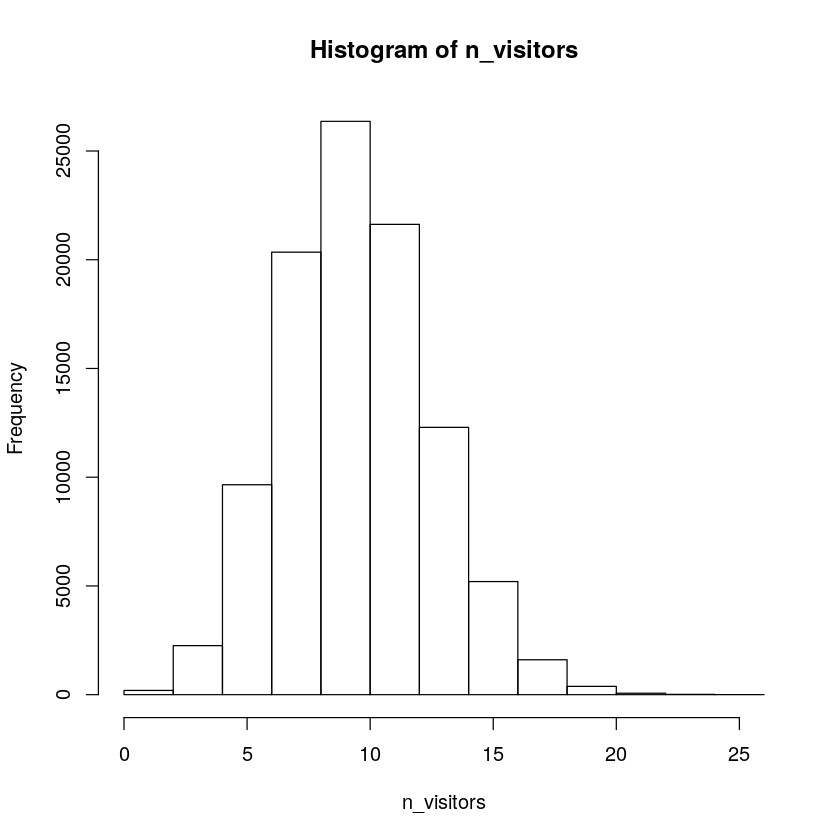In :
# Probability of 5 or more vistors
sum(n_visitors >= 5) / length(n_visitors)

0.97551

# Adding a prior to the model¶

We're not so sure that your ad will get clicked on exactly 10% of the time. Instead of assigning proportion_clicks a single value we are now going to assign it a large number of values drawn from a probability distribution.

For now, we are going to assume that it's equally likely that proportion_clicks could be as low as 0% or as high as 20%. These assumptions translate into a uniform distribution.

In :
n_samples <- 100000

# Update proportion_clicks
proportion_clicks <- runif(n = n_samples, min = 0.0, max = 0.2)

n_visitors <- rbinom(n = n_samples, size = n_ads_shown, prob = proportion_clicks)

# Visualize proportion clicks
hist(proportion_clicks)

# Visualize n_visitors
hist(n_visitors)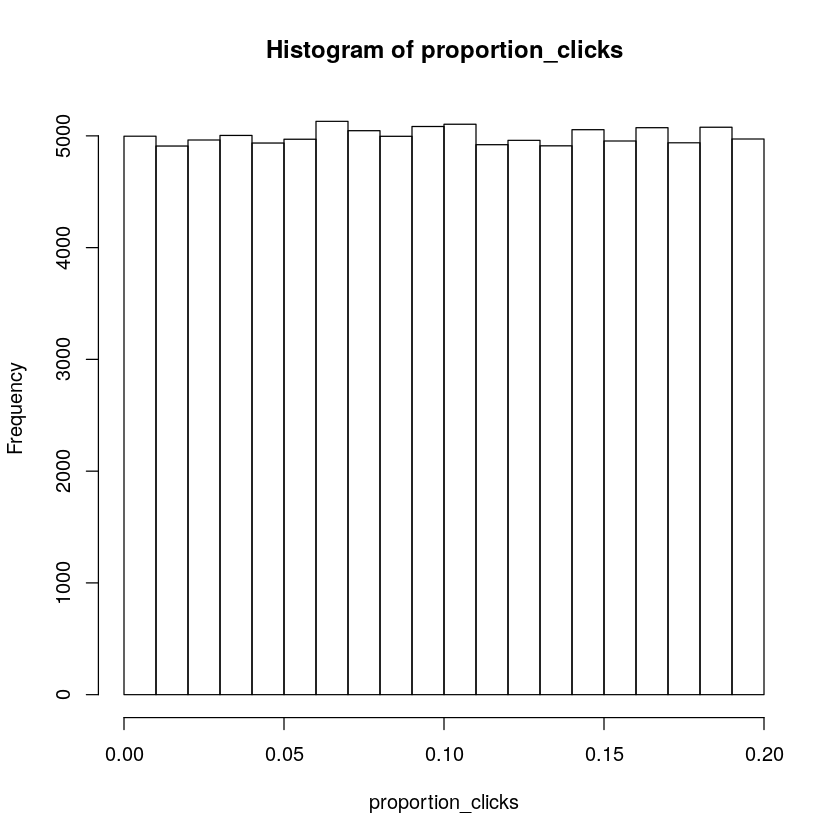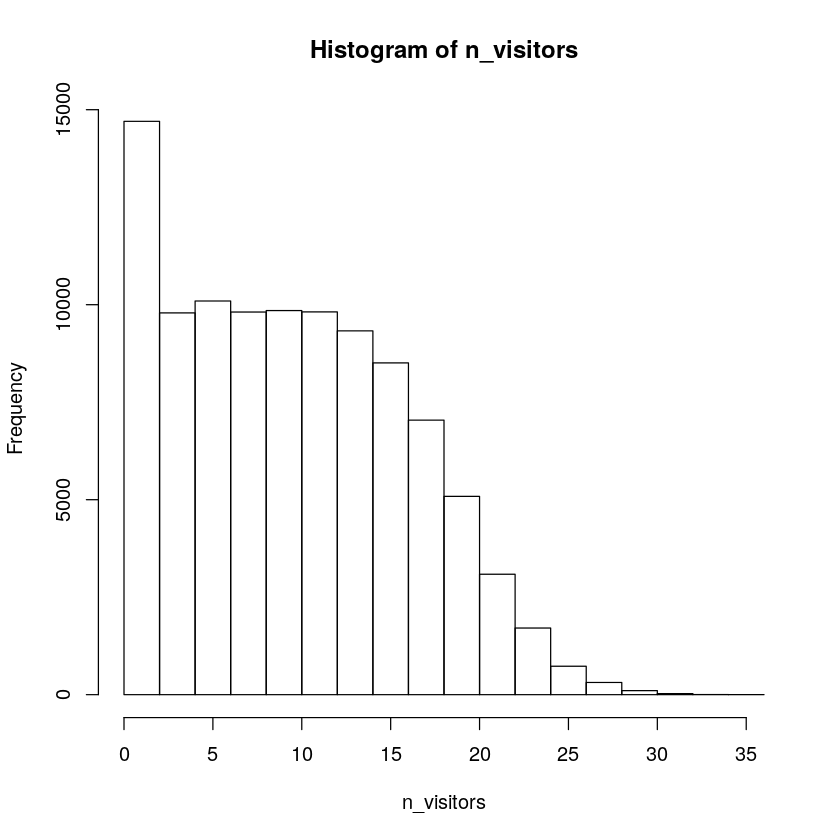This looks very different from the histogram of n_visitors we got when proportion_clicks was exactly 0.1. With the added uncertainty in proportion_clicks the uncertainty over the number of visitors we 'll get also increased.

In :
# Probability of 5 or more vistors
sum(n_visitors >= 5) / length(n_visitors)

0.75508

## Update a Bayesian model with data¶

You ran your ad campaign, and 13 people clicked and visited your site when the ad was shown a 100 times. You would now like to use this new information to update the Bayesian model.

The model we put together previously resulted in two vectors: (1) proportion_clicks that represents the uncertainty regarding the underlying proportion of clicks and (2) n_visitors which represents the uncertainty regarding the number of visitors you would get.

In :
# Create the prior data frame
prior <- data.frame(proportion_clicks, n_visitors)

# Examine the prior data frame

A data.frame: 6 × 2
proportion_clicksn_visitors
<dbl><int>
10.1304072310
20.1788409912
30.12716597 9
40.03829120 5
50.1385943014
60.07483191 8
In :
p = ggplot(prior, aes(n_visitors, proportion_clicks)) + geom_point(alpha=0.3)
ggMarginal(p, type = "histogram")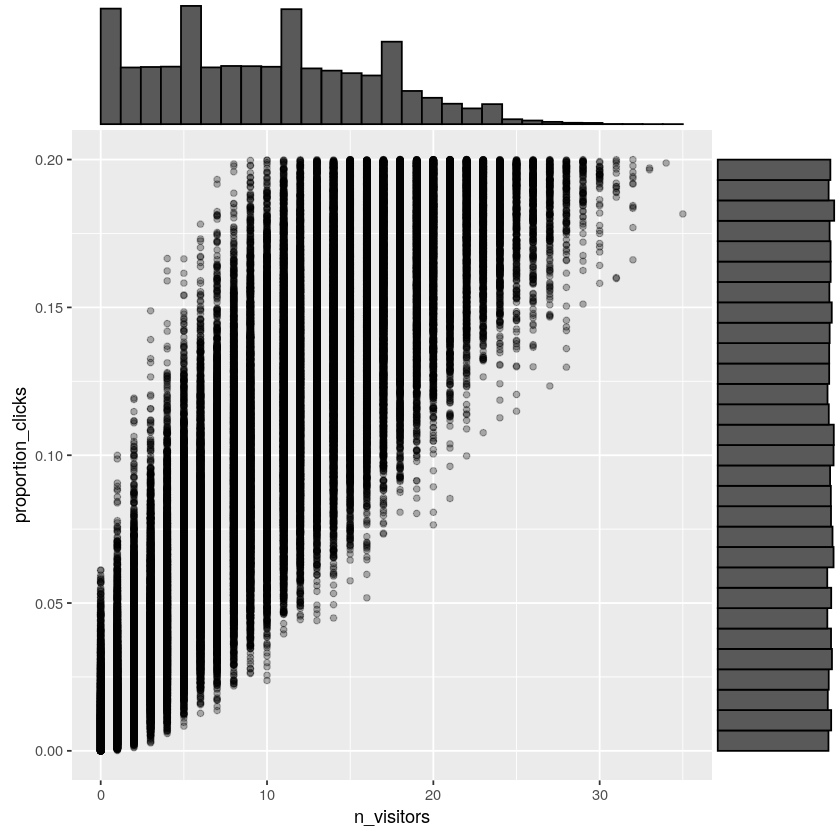The reason we've called it prior is because it represents the uncertainty before (that is, prior to) having included the information in the data.

prior$n_visitors represented the uncertainty over how many visitors you would get because of the ad campaign. But now you know you got exactly 13 visitors. We update prior to include this information by conditioning on this data. That is, filtering away all rows where prior$n_visitors isn't equal to 13.

In :
# Create the posterior data frame
posterior <- prior[prior$n_visitors == 13, ] # Visualize posterior proportion clicks hist(posterior$proportion_clicks)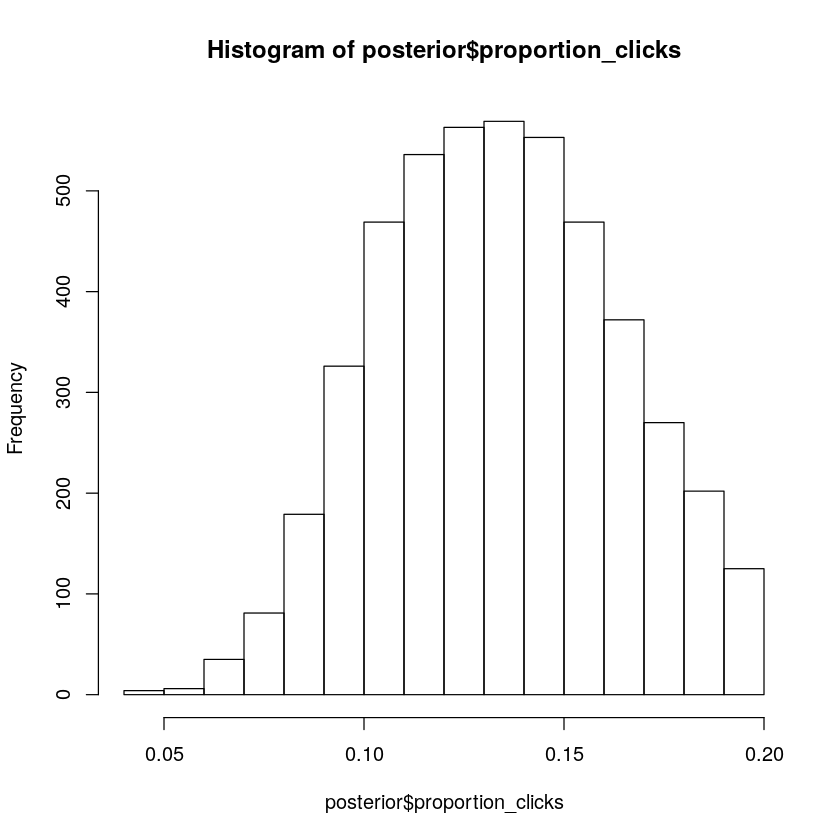# How many visitors could your site get?¶

Previously we updated the probability distribution over the underlying proportions of clicks (proportion_clicks) using new data. Now we want to use this updated proportion_clicks to predict how many visitors we would get if we reran the ad campaign.

In :
# Assign posterior to a new variable called prior
prior <- posterior

A data.frame: 6 × 2
proportion_clicksn_visitors
<dbl><int>
960.139078213
1360.132943813
1460.148245413
1810.116126913
1990.125244213
2000.114958013

This will represent the uncertainty regarding the new ad campaign you haven't run yet.

While proportion_clicks represents the uncertainty regarding the underlying proportion of clicks for the next ad campaign, n_visitors has not been updated yet.

In :
# Replace prior$n_visitors with a new sample and visualize the result n_samples <- nrow(prior) n_ads_shown <- 100 prior$n_visitors <- rbinom(n_samples, size = n_ads_shown, prob = prior$proportion_clicks) hist(prior$n_visitors)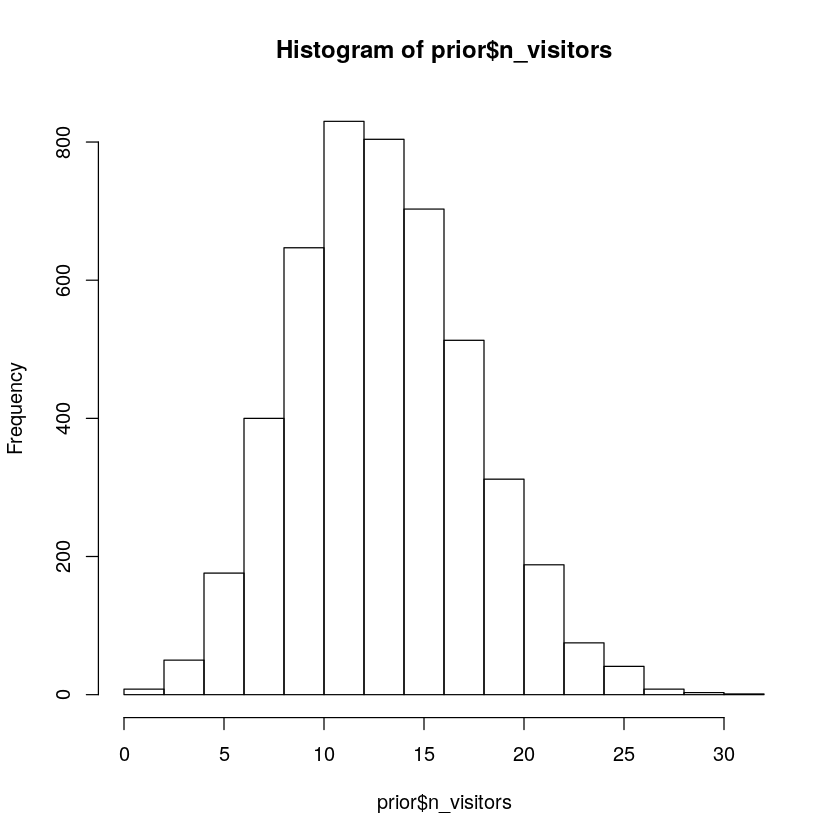The plot shows a probability distribution over the number of site visitors a new ad campaign would bring in. It now looks pretty likely that there would be more than, say, 5 visitors because of the campaign.

In :
# Calculate the probability that you will get 5 or more visitors
sum(prior$n_visitors >= 5) / length(prior$n_visitors)

0.987812565665056

# Explore using the Beta distribution as a prior¶

The Beta distribution is a useful probability distribution when you want model uncertainty over a parameter bounded between 0 and 1. Here we will explore how the two parameters of the Beta distribution determine its shape.

In :
# Explore using the rbeta function
beta_sample <- rbeta(n = 1000000, shape1 = 1, shape2 = 1) # Beta(1,1)

# Visualize the results
hist(beta_sample)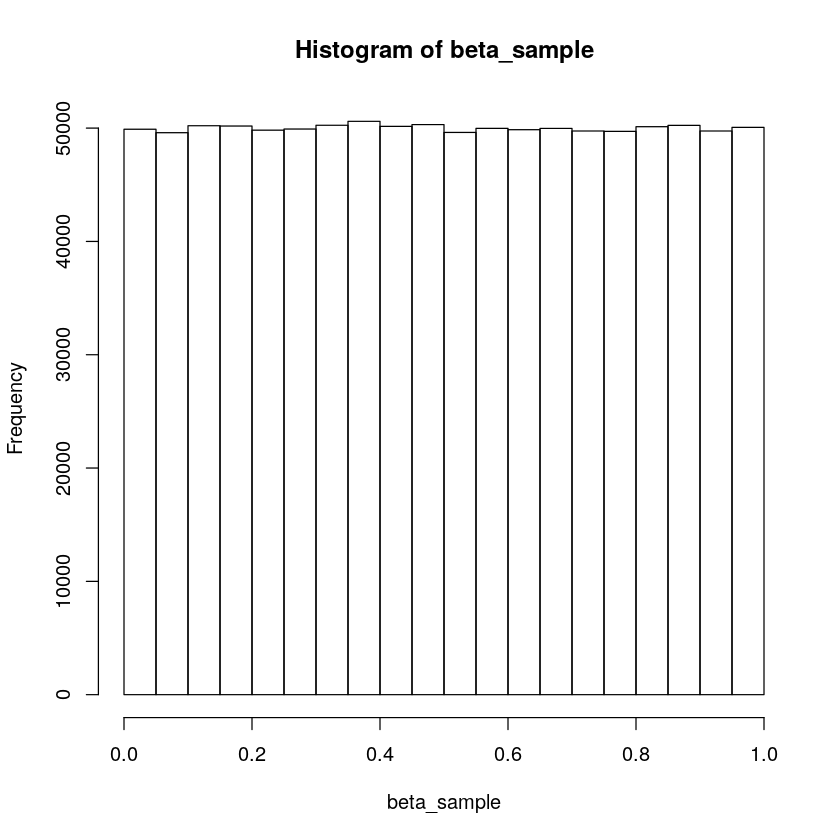A Beta(1,1) distribution is the same as a uniform distribution between 0 and 1. It is useful as a so-called non-informative prior as it expresses that any value from 0 to 1 is equally likely.

In :
# Modify the parameters
beta_sample <- rbeta(n = 1000000, shape1 = 100, shape2 = 100)

# Visualize the results
hist(beta_sample)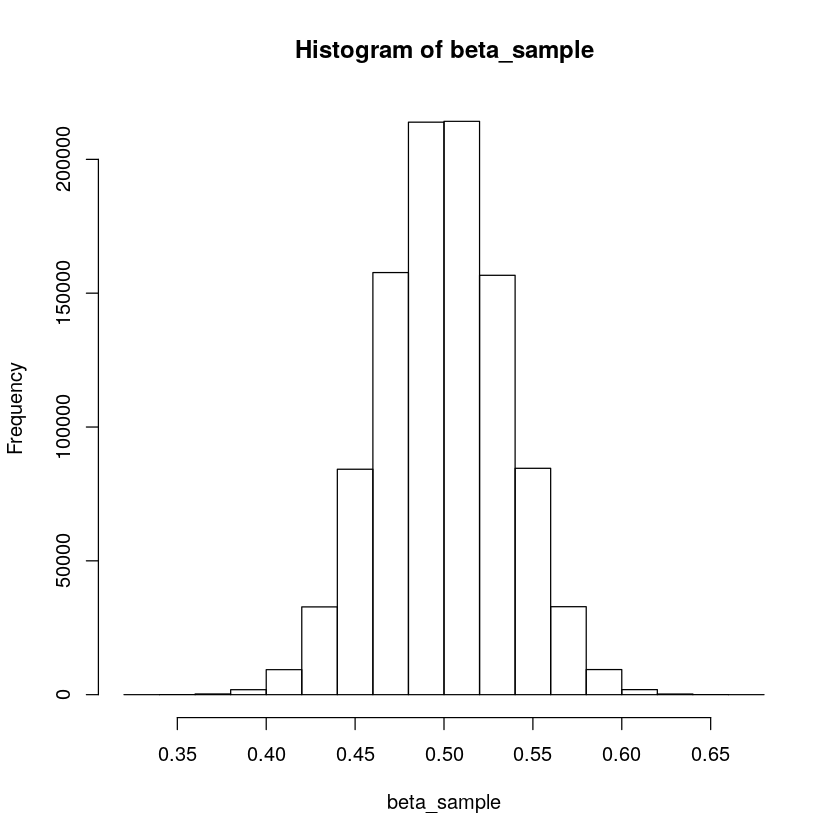The larger shape parameters are, the more concentrated the beta distribution becomes. When used as a prior, this Beta distribution encodes the information that the parameter is most likely close to 0.5.

In :
# Modify the parameters
beta_sample <- rbeta(n = 1000000, shape1 = 100, shape2 = 20)

# Visualize the results
hist(beta_sample)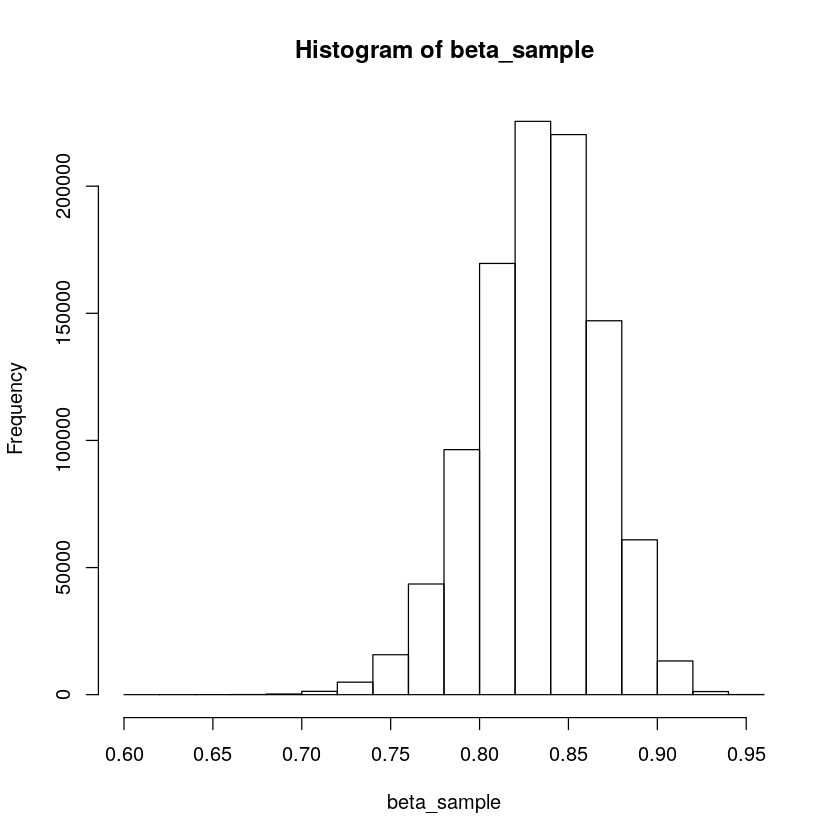The larger the shape1 parameter is the closer the resulting distribution is to 1.0 and the larger the shape2 the closer it is to 0.

In :
# Modify the parameters
beta_sample <- rbeta(n = 1000000, shape1 = 10, shape2 = 150)

# Visualize the results
hist(beta_sample)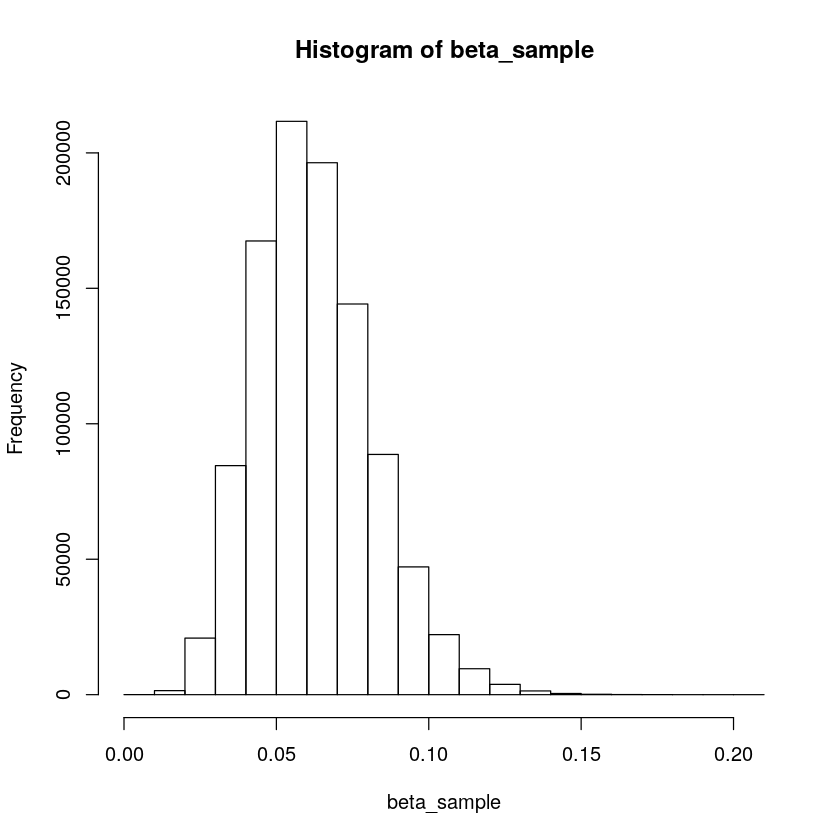# Pick the prior that best captures the information¶

In :
beta_sample1 <- rbeta(n = 10000, shape1 = 3, shape2 = 12)
beta_sample2 <- rbeta(n = 10000, shape1 = 15, shape2 = 75)
beta_sample3 <- rbeta(n = 10000, shape1 = 5, shape2 = 95)
beta_sample4 <- rbeta(n = 10000, shape1 = 30, shape2 = 10)

figure1 <- multi_panel_figure(columns = 2, rows = 2, panel_label_type = "none")

q1 <- ggplot() + geom_histogram(aes(beta_sample1), bins=40, colour="black", fill="blue", alpha=0.5) +
theme_joy() + xlim(c(0,1))

q2 <- ggplot() + geom_histogram(aes(beta_sample2), bins=40, colour="black", fill="green", alpha=0.5) +
theme_joy() + xlim(c(0,1))

q3 <- ggplot() + geom_histogram(aes(beta_sample3), bins=40, colour="black", fill="red", alpha=0.5) +
theme_joy() + xlim(c(0,1))

q4 <- ggplot() + geom_histogram(aes(beta_sample4), bins=40, colour="black", fill="yellow", alpha=0.5) +
theme_joy() + xlim(c(0,1))


Most ads get clicked on 5% of the time, but for some ads it is as low as 2% and for others as high as 8%.

There are many different probability distributions that one can argue captures this information. Out of the four Beta distribution shown below, which captures this information the best?

In :
figure1 %<>%
fill_panel(q1, column = 1, row = 1) %<>%
fill_panel(q2, column = 2, row = 1) %<>%
fill_panel(q3, column = 1, row = 2) %<>%
fill_panel(q4, column = 2, row = 2)
figure1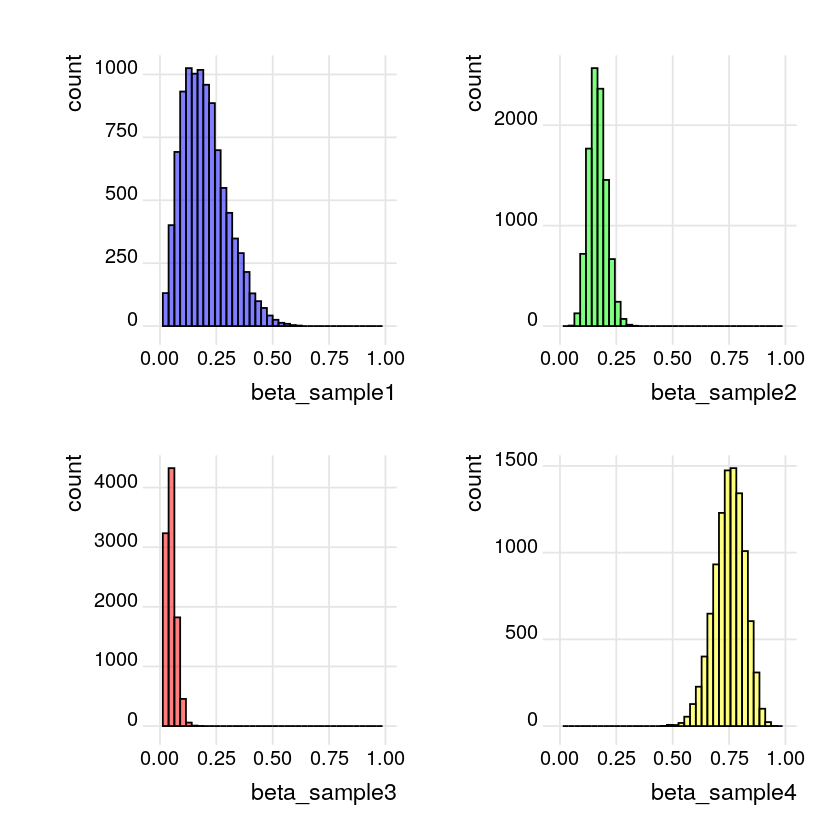The best distribution would be beta_sample3 rbeta(shape1 = 5, shape2 = 95)

## Original Model¶

In :
n_draws <- 100000

proportion_clicks <- runif(n_draws, min = 0.0, max = 0.2)
n_visitors <- rbinom(n_draws, size = n_ads_shown, prob = proportion_clicks)

prior <- data.frame(proportion_clicks, n_visitors)
posterior <- prior[prior$n_visitors == 13, ] # This plots the prior and the posterior in the same plot par(mfcol = c(2, 1)) hist(prior$proportion_clicks,
xlim = c(0, 0.25))
hist(posterior$proportion_clicks, xlim = c(0, 0.25))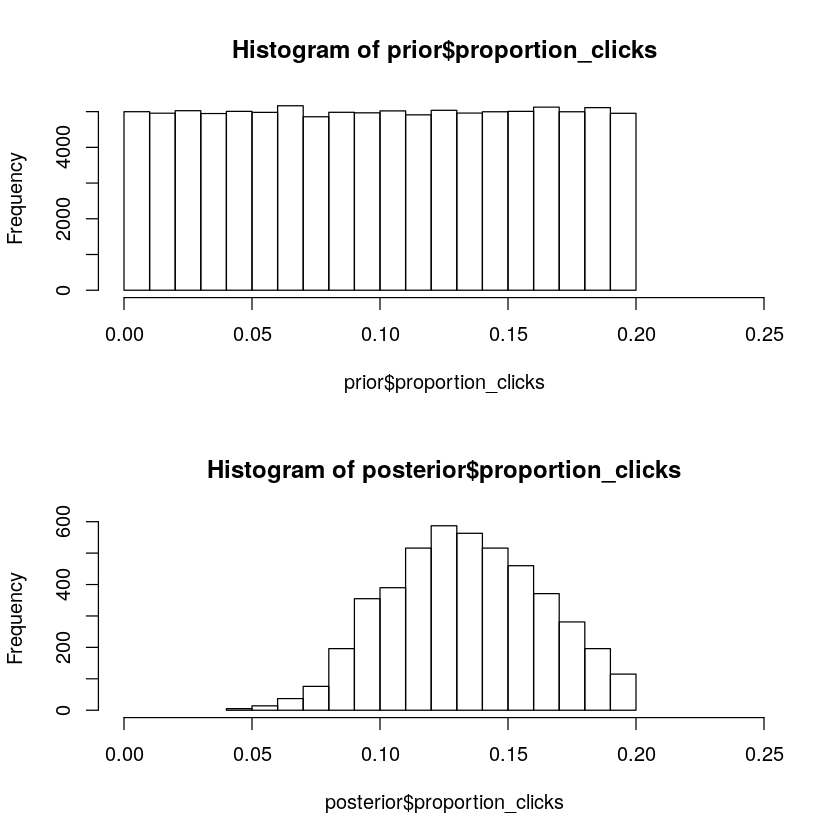## Change the model to use an informative prior¶ In : n_draws <- 100000 n_ads_shown <- 100 # Change the prior on proportion_clicks proportion_clicks <- rbeta(n_draws, shape1 = 5, shape2 = 95) n_visitors <- rbinom(n_draws, size = n_ads_shown, prob = proportion_clicks) prior <- data.frame(proportion_clicks, n_visitors) posterior <- prior[prior$n_visitors == 13, ]

# This plots the prior and the posterior in the same plot
par(mfcol = c(2, 1))
hist(prior$proportion_clicks, xlim = c(0, 0.25)) hist(posterior$proportion_clicks,
xlim = c(0, 0.25))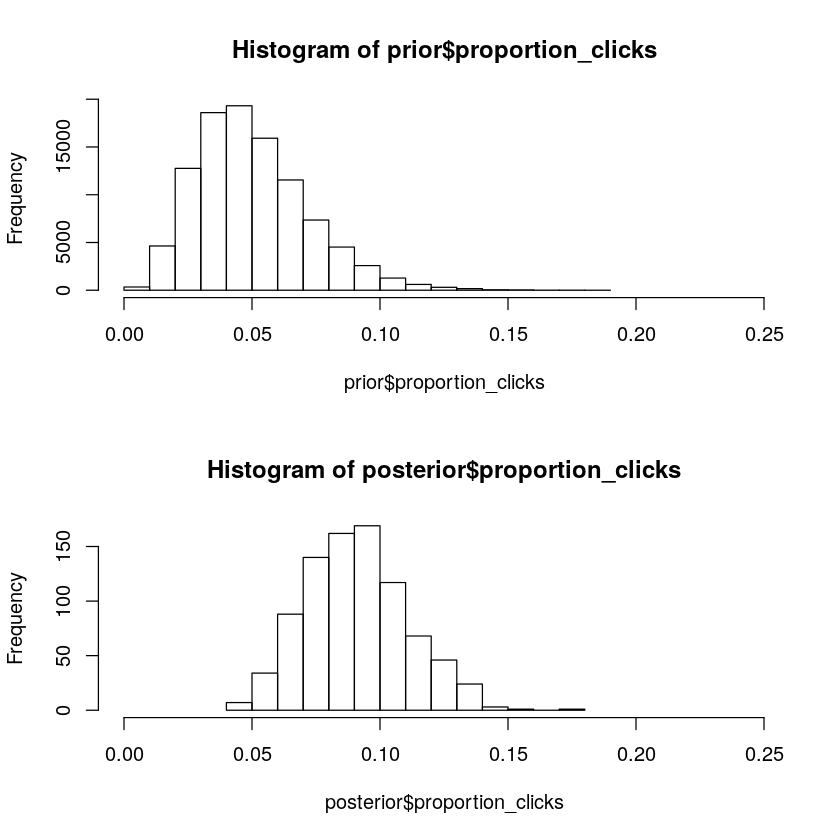# Fit the model using another dataset¶

Let's fit the binomial model to both the video ad data (13 out of 100 clicked) and the new text ad data (6 out of a 100 clicked).

In :
# Define parameters
n_draws <- 100000

proportion_clicks <- runif(n_draws, min = 0.0, max = 0.2)
n_visitors <- rbinom(n = n_draws, size = n_ads_shown, prob = proportion_clicks)

prior <- data.frame(proportion_clicks, n_visitors)

# Create the posteriors for video and text ads
posterior_video <- prior[prior$n_visitors == 13, ] posterior_text <- prior[prior$n_visitors == 6, ]

# Visualize the posteriors
hist(posterior_video$proportion_clicks, xlim = c(0, 0.25)) hist(posterior_text$proportion_clicks, xlim = c(0, 0.25))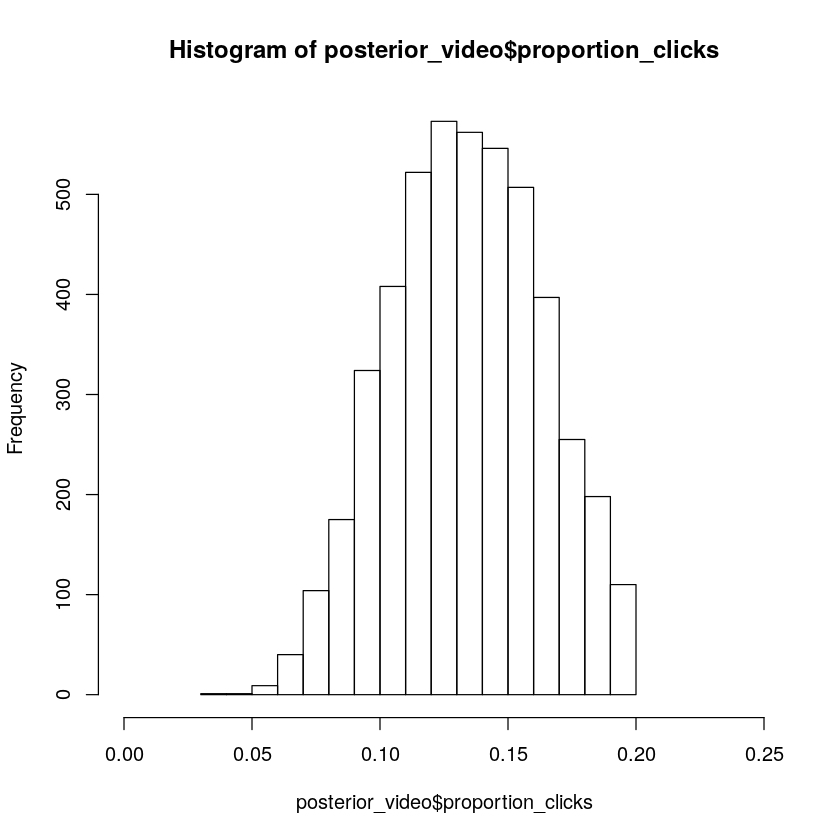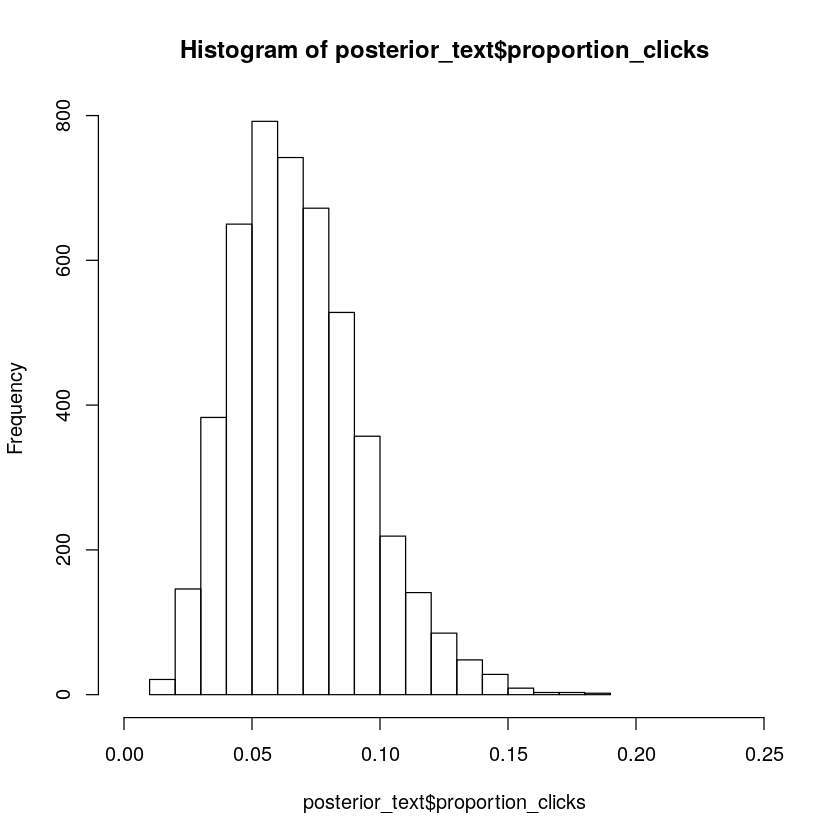## Calculating the posterior difference¶

The posterior proportion_clicks for the video and text ad has been put into a single posterior data frame. The reason for [1:4000] is because these proportion_clicks are not necessarily of the same length, which they need to be when put into a data frame.

Now it's time to calculate the posterior probability distribution over what the difference in proportion of clicks might be between the video ad and the text ad.

In :
posterior <- data.frame(
video_prop = posterior_video$proportion_clicks[1:4000], text_prop = posterior_text$proportion_click[1:4000])

# Calculate the posterior difference: video_prop - text_prop
posterior$prop_diff <- posterior$video_prop - posterior$text_prop # Visualize prop_diff hist(posterior$prop_diff, xlim = c(-0.15, 0.2))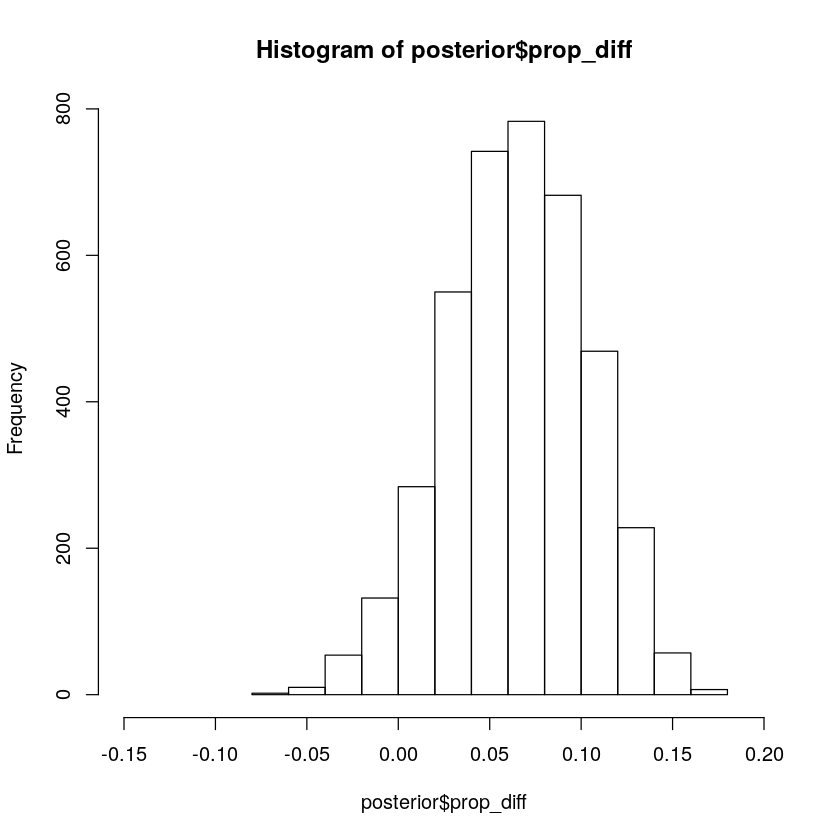In [ ]:
# Calculate the median of prop_diff
median(posterior$prop_diff)  Calculate the probability that proportion of clicks is larger for the video ad than for the text ad. That is, calculate the proportion of samples in posterior$prop_diff that are more than zero.

In :
# Calculate the proportion
sum(posterior$prop_diff > 0) / length(posterior$prop_diff)

0.9505

It's pretty likely that the video ad is better.

## A small decision analysis 1¶

Each visitor spends $2.53 on average, a video ad costs $0.25 and a text ad costs \$0.05. Let's figure out the probable profit when using video ads and text ads!

In :
visitor_spend <- 2.53